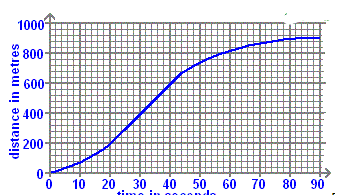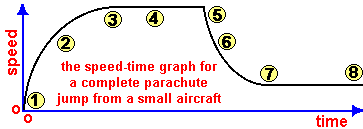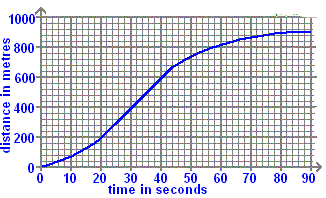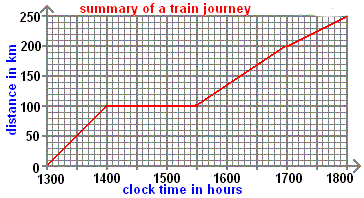# Combined quiz on forces and their effects and speeding up

#### Quiz Description

In this quiz, we will be looking at forces and their effects and speeding up. This quiz will help you understand how forces cause motion, and this motion is usually through a particular distance. Forces are one of the very few important topics in Physics, and a very fertile one for the exam and test settings. So this quiz is a very good quiz if you want to have a very good mark or pass during your tests and exams.

Forces cause motion, stop motion, and change the direction of motion of a moving object. When a force is applied, an effect is speeding up the object, then Newton’s law is in play. Here, the Force is the product of mass and acceleration(which is the speed in a particular direction per unit time). Mathematically,
F=ma
Note that, speeding up simply means acceleration.

In our quizzes, we test you to be up to the task, and for this quiz, students will be tested based on practical situations so for you to be familiar with the questions that could be asked in your exam or so, you have to take the quiz. Other Physics quizzes are available too.
Good luck!

A racing car is travelling at an average speed of 40 m/s through a curvey section of the race track. How long will it take the driver to cover 1 km?

Correct
•  60 s
•  50 s
• 25 s
• 40 s

You are given the following masses and volumes for various blocks of materials. The mass of 1 cm3 of water is 1 g. From them choose which one will float on water?

Correct
•  material Z: mass = 20 g, volume = 19 cm3
•     material W: mass = 12 g, volume = 10 cm3
•  material Y: mass = 27 g, volume = 30 cm3
•  material X: mass = 22 g, volume = 20 cm3

Which of the following matches a speed of 9.8m/s?

Correct
•  a sprinter runs 100m in 10.2s
•  a cyclist covers 500m in 40s
•  a car moves 400m in 36s
•  a conveyer belt moves along 20m in 2.5s

Which of the following are units of mass?

Correct
• grams per centimetre cubed
• kilogram
• centimetre cubed
• newton

Which of the following is a unit of force?

Correct
• N
• m
•  m/s
• s

Which of the following are units of volume?

Correct
• kilogram
• grams per centimetre cubed
• newton
•  centimetre cubed

Which of the following is a unit of speed?

Correct
• newtons
•  newton x metres
•  metres per sec per sec
•  metres per second

A train travels 40 km in 20 minutes. What is the average speed of the train?

Correct
•  20 km/hour
• 120 km/hour
•  80 km/hour
•  2 km/hour

Which of the following are units of density?

Correct
• grams per centimetre cubed
• newton
• kilogram
• centimetre cubed

Which is NOT true about the situation illustrated?

Correct
•  if the cyclist crouches down and wears smoother clothing the speed cannot be increased without increasing pedal power
•  if the pedal power force exceeds the drag or friction forces, the cyclist will increase in speed
•  when the pedal power force equals the drag or friction forces, the speed is constant
•  drag or air resistance is caused by the collision of air particles with the surface of the moving object

Which of the following are units of mass?

Correct
• N
• kg
•  g/cm3
• cm3

The graph shows part of an urban car journey in terms of the distance the car has travelled in a certain time. How far has the car travelled between 0 and 25 seconds?Correct
• 320 m
•  300 m
• 340 m
•  260 m

The graph shows how the speed of a parachutist varies from point (1) immediately jumping out of an aeroplane, to landing safely with the parachute opened at point (8). Between which points is the air resistance force (friction) equal to the force of gravity on the parachutist?Correct
•   points (3) and (5)
•  points (5) and (6)
•  points (1) and (3)
• points (6) and (7)

The graph shows part of an urban car journey in terms of the distance the car has travelled in a certain time. The car engine produces the forward motion thrust force F1. Acting against this are the combined forces of (i) moving parts friction (including braking) and (ii) the drag from air resistance, giving a total force F2. In which time span is force F2 more than F1?Correct
•  82 to 90 seconds
•  44 to 82 seconds
•  20 to 44 seconds
•  0 to 20 seconds

At what time has the train travelled 215 km?Correct
•  5.30pm
•  5.15pm
• 5.45pm
• 5pm